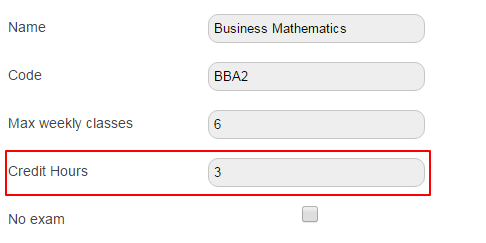The formula for calculation of GPA or Grade point Average is as shown below :

Sum of (Credit Hours assigned to the Subject × Credit Points corresponding to the grade secured by the student)

-----------------------------------------------------------------------------------------------------

Sum of credit hours of all the subjects in a batch

ExampleCode Subject Credit Hours Grade Received Batch 1 BBA1 Financial Accounting 3 B BBA2 Business Mathematics 3 B+Suppose ‘B’ has credit points 3 and ‘B+’ 3.5

Total Credit Points Earned for Financial Accounting = Credit Points × Credit Hours

= 3 × 3 = 9

Total Credit Points Earned for Business Mathematics = Credit Points × Credit Hours

= 3 × 3.5 = 10.5

GPA = Sum of (Credit Points × Credit Hours)/Sum of Credit Hours

= (10.5 + 9) / 6 = 3.25